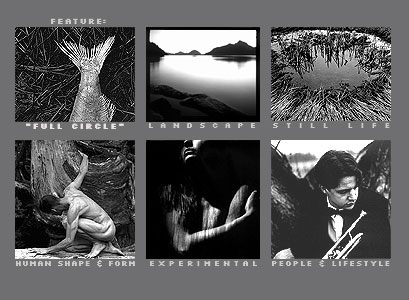P R O F I L EG A L L E R YO R D E RC O N T A C T    I N F OG  A  L  L  E  R  Y>   >      "I am drawn to simplicity. I am held by energy; an energy to       capture, and in a million grains of silver render it alive once       more."       r o b e r t   j.  w i l k i n s o n >  F E A T U R E D   E X H I B I T :   "F U L L   C I R C L E "  >  L A N D S C A P E  > P E O P L E   &   L I F E S T Y L E  > H U M A N   S H A P E   &   F O R M  > S T I L L    L I F E  > E X P E R I M E N T A LJanuary 2005 - The " F E A T U R E D   E X H I B I T " is an exhibition of fish images entitled: " F U L L  C I R C L E " In the exhibitions, click on thumbnails to bring up an enlargement. To reduce the download time, the quality of the images has had to be greatly reduced compared to the originals. All work is for sale. See the order page for details. Contact me for more information: rob@robertjwilkinson.com >  S E N D   T H I S   S I T E   T O   A   F R I E N DT O P© 2001 - 2005   R o b e r  t   J   W i l k i n s o n             P r i v a c y   P o l i c ys i t e   d e s i g n